# MEDIAN Function Examples in Excel, VBA, & Google Sheets

This tutorial demonstrates how to use the Excel MEDIAN Function in Excel to calculate the median number.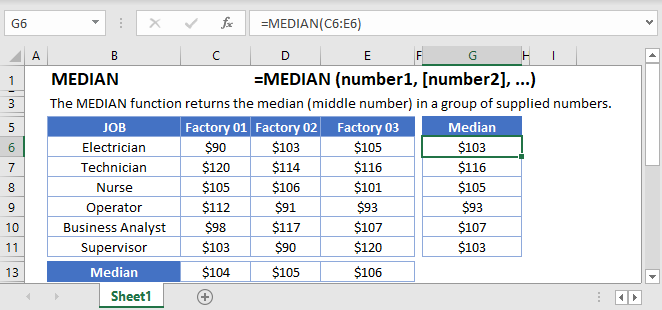## MEDIAN Function Overview

The MEDIAN Function Calculates the median number.

To use the MEDIAN Excel Worksheet Function, select a cell and type: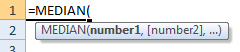(Notice how the formula inputs appear)

### MEDIAN function Syntax and inputs:

``=MEDIAN (number1, [number2], ...)``

number1 – A number or cell reference that refers to numeric values.

number2 – [optional] A number or cell reference that refers to numeric values

## What is the Median?

In statistical analysis, the median is the middle data point in a series of data. It reflects the central tendency in a group data. If you have 7 numbers, the median number would be the 4th largest number.

Let’s look at an example of student test scores. There are 7 students, so the median score is the 4th best score (Pam’s)

``=MEDIAN(C3:C9)``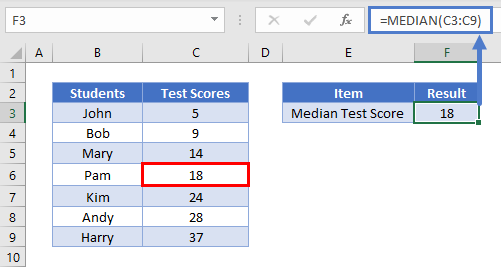If you have an even number of data points (where this is not a middle data point), the median number is the average of the two central-most points. Returning to our student test score example, if you have 6 data points, the median would be the average of the 3rd and 4th best score.

``=MEDIAN(C3:C9)``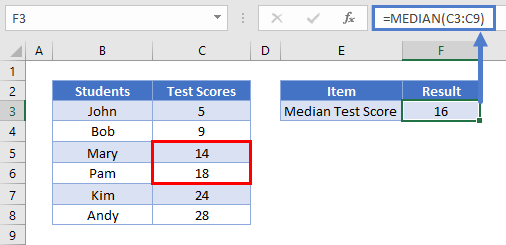## Median vs. Mean

As discussed above, the median is the middle number in a data series. The mean is the average number out of a data series. Both numbers represent the central tendency of a data set, but which is the most appropriate to use?

A good rule of thumb is if you have outlier data, then the median will be more representative of the data than the mean (average).

### What is outlier data?

Outlier data are extreme data points that distort the value of the mean. Mean is very sensitive to outlier data hence the distortion.

For example, you want to find out the median cost of five different laptops.

``=MEDIAN(C3:C7)``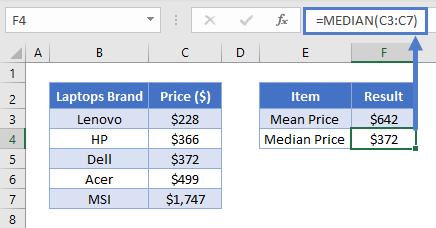You will notice that mean & median values are not close. It is because of the outlier data point: \$1747. In this case, the median value might be more representative of laptop prices because the mean is so heavily weighted by the outlier price.

## How to use the MEDIAN Function

To use the Excel MEDIAN Function, simply enter your desired data range into the function:

``=MEDIAN(C3:C7)``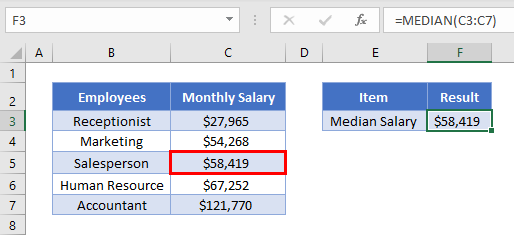Notice how with an odd number of data points the MEDIAN Function returns the middle number?

Now look at how the MEDIAN Function averages the middle numbers when there is an even number of data points:

``=MEDIAN(C3:C8)``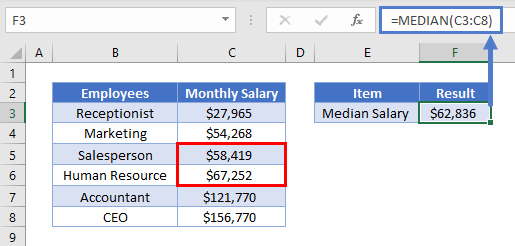### Easy Excel Automation## Empty Cells or Cells With Text

The MEDIAN Function ignores cells that are empty or that contain non-numerical values.

Important: The MEDIAN Function will ignore numbers stored as text. To use the MEDIAN Function with numbers stored as text, first, use the VALUE Function to convert the numbers stored as text to actual numbers.

``=VALUE(B3)``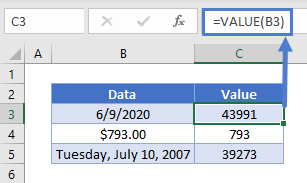## Median Date

Excel store dates as serial numbers.

``=VALUE(B3)``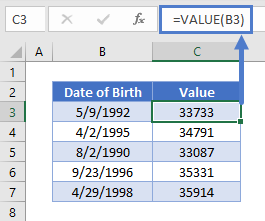This makes it easy to calculate the median date.

``=MEDIAN(C3:C7)``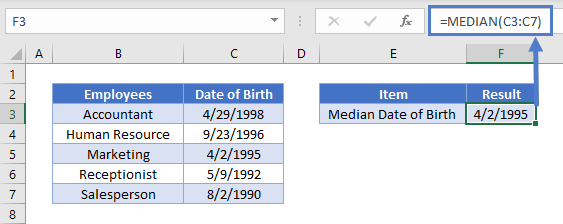## Median Age

Using this knowledge, we can also calculate the median age.

First, we will calculate the median date of birth.

``=MEDIAN(C3:C7)``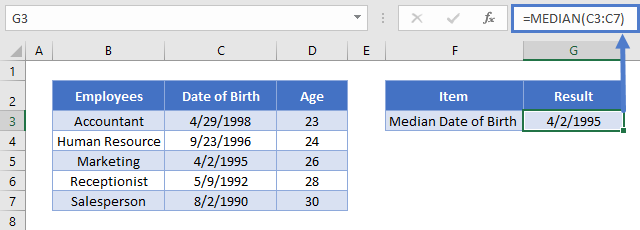Next, we will use the YEARFRAC Function to calculate the median age:

``=MEDIAN(D3:D7)``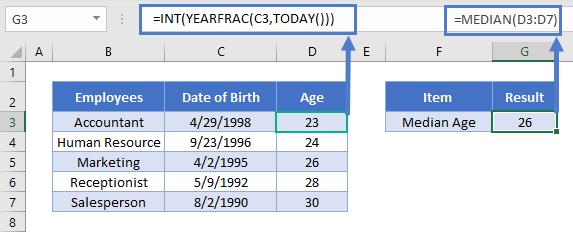## Median If

So far we’ve been calculating the median of all numbers in a range; this is how the MEDIAN Function works.

But what if you want to calculate the median of only a subset of data?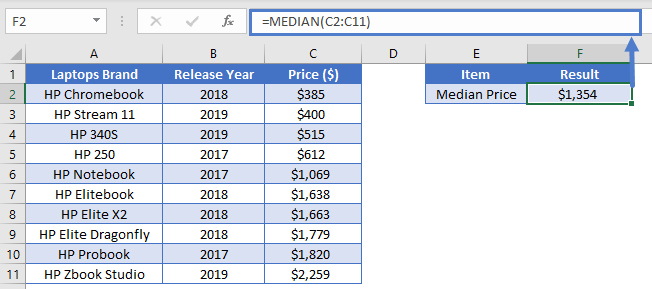Let’s say you want to calculate the average (mean) & median price the HP laptops that were released in 2017

Excel has an AVERAGEIFS Function that allows you to take the average of a subset of numbers that meet a certain condition. Average of HP laptops that were released in 2017 can be easily calculated using the AVERAGEIF function.

``=AVERAGEIF(\$B\$2:\$B\$11, \$E2, \$C\$2:\$C\$11)``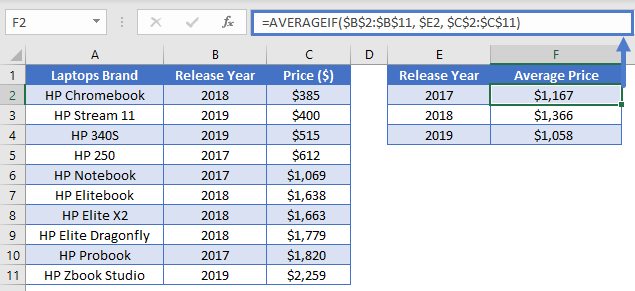However, Excel doesn’t have a corresponding “median if” function. You can create your own “median if” with an Array formula containing the MEDIAN and IF Functions.

This is the generic formula to calculate “median if

=MEDIAN(IF(criteria_range=criteria, median_range))

Excel 2019 and Earlier: After entering the formula, instead of pressing ENTER, you must press CTRL + SHIFT + ENTER. This turns the formula into an array. You can identify arrays by the curly brackets surrounding the formula

{=MEDIAN(IF(criteria_range=criteria, median_range))}

Important: You can not manually type in the curly brackets, this will not work. You must use CTRL + SHIFT + ENTER

Excel 365 and Newer Versions of Excel: After the release of Excel 2019, Microsoft changed how array formulas work. Now, you no longer need to use CTRL + SHIFT + ENTER. You can simply enter the formula like normal.

Returning to our example, we will use the following formula:

``{=MEDIAN(IF(\$B\$2:\$B\$11 = \$E2, \$C\$2:\$C\$11))}``

Excel will look for 2017 in “Release Year” range and return only those values to the MEDIAN function. Hence, calculating only the median of a subset of our data.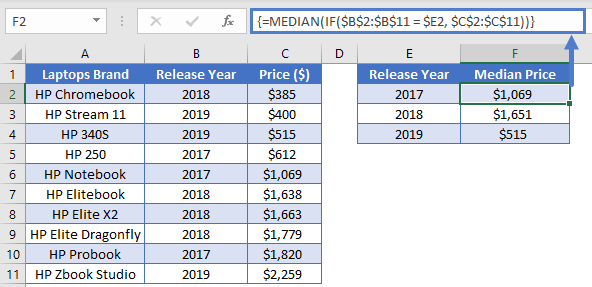Read our Median If Tutorial to learn more about how to calculate the “median if”, including details about how the array function actually works and how to calculate the median with multiple criteria.

Note: The above formula uses absolute references (the \$ signs) to lock cell references when copying formulas. If you aren’t familiar with this, please read our Excel References Guide.

## MEDIAN Function in Google Sheets

The MEDIAN function works exactly the same in Google Sheets as in Excel: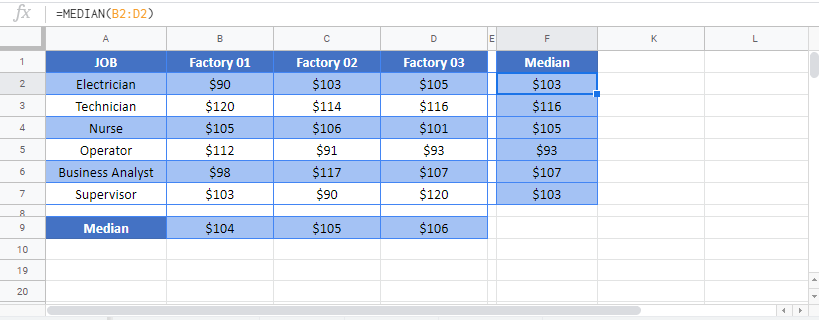## MEDIAN Examples in VBA

You can also use the MEDIAN function in VBA. Type:

``application.worksheetfunction.median(number1,number2)``

Executing the following VBA statements

``````Range("B2") = Application.WorksheetFunction.Median(Range("A2:A9"))
Range("B3") = Application.WorksheetFunction.Median(Range("A3:A9"))
Range("B4") = Application.WorksheetFunction.Median(Range("A4:A9"))
Range("B5") = Application.WorksheetFunction.Median(Range("A5:A9"))
Range("B6") = Application.WorksheetFunction.Median(Range("A6:A9"))
Range("B7") = Application.WorksheetFunction.Median(Range("A7:A9"))
Range("B8") = Application.WorksheetFunction.Median(Range("A8:A9"))
Range("B9") = Application.WorksheetFunction.Median(Range("A9:A9"))``````

will produce the following results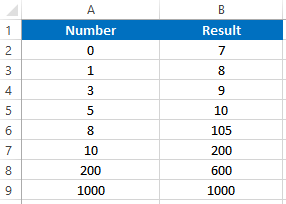For the function arguments (array, etc.), you can either enter them directly into the function, or define variables to use instead.